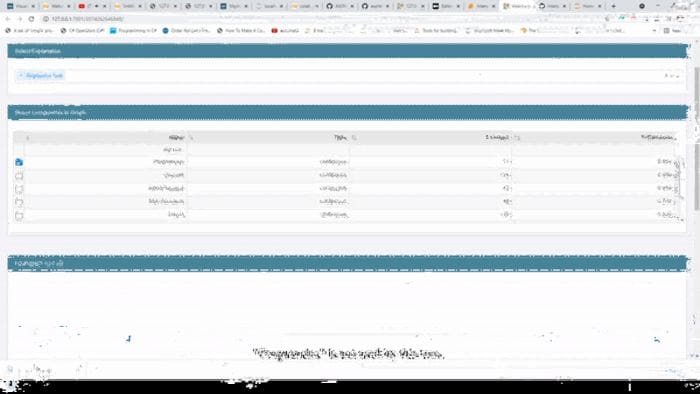# Dashboards for Interpreting & Comparing Machine Learning Models

This article discusses using Interpret to create dashboards for machine learning models.

By Himanshu Sharma, Bioinformatics Data AnalystSource: By Author

With the advent of technology, there are multiple machine learning algorithms in the Data Science field which makes it really difficult for a User/Data Scientist/ML Engineer to select the best model according to the dataset that they are working on.

Comparing different models can be one way of selecting the best model, but it is time taking process where we will create different machine learning models and then compare their performance. It is also not feasible because most of the models are black-box and we don’t know what is going on inside the model and how it will behave. In short, we don’t know how to interpret the model because of the model complexity and model being black-box.

Without interpreting the models it is difficult to understand how a model is behaving and how it will behave on the new data provided to it. It not only helps us understand the model predictions but also helps us understand how it reached that particular prediction.

The next big problem is how to interpret these models and their behavior, let me bring light to this. What if I tell you that you can not only interpret different models but also compare their performances? Yes, that's right now we can see inside the black box and understand what the model is doing.

Interpret is an open-source python library that is used to interpret and analyze the performance of different machine learning models. In this article, we will see how to use interpret for model interpretation and visualizing model performance. There is something really cool that we will explore at the end of this article and I am sure that is something which is really helpful for all Data Scientists/ML Engineers etc. so stay tuned.

Let’s get started…

### Installing Required Libraries

Like any other python library, we will install Interpret using pip installation. The command given below will perform the installation.

``pip install interpret``

### Importing Required Libraries

Next, we will import all the required libraries that will be used in this article. We will load Pandas, Interpret, etc.

``````import pandas as pd
from interpret import show
from interpret.glassbox import RegressionTree
from sklearn.model_selection import train_test_split``````

We will import other functionalities of Interpret as and when required.

### Creating Machine Learning Model

Now we will create a Machine Learning model which we will interpret using interpret library. As you can see we have already imported the library for loading the data and for splitting it also, so let’s start by loading the data first and then create the model. The dataset we will use here is the famous Diabetes dataset.

``````df = pd.read_csv("Diabetes.csv")
X_train, X_test, y_train, y_test = train_test_split(df[['Pregnancies', 'Glucose','BloodPressure','SkinThickness','Insulin','BMI','DiabetesPedigreeFunction','Age']], df['Outcome'], test_size=0.3)#Creating Model
reg_tree = RegressionTree(random_state=42)
reg_tree.fit(X_train, y_train)``````

We will create more models later so that we can compare different models.

### Interpreting & Performance Analysis using Visualization

Now we will interpret the models and also watch the performance of the model.

``````#global interpretation
reg_tree_global = reg_tree.explain_global(name='Regression Tree')
show(reg_tree_global)``````Source: By Author

This is the overall performance of the model, you can select different features from the drop-down to see how it is used in the model. This is the global interpretation of the model for local interpretation(Individual predictions) we can use the local function.

Now let us visualize the performance of the model.

``reg_tree_perf = RegressionPerf(reg_tree.predict).explain_perf(X_test, y_test, name='Regression Tree')show(reg_tree_perf)``Performance(Source: By Author)

### Bonus

Here comes the interesting part, in the bonus section I will show you how you can create a model dashboard containing the interpretation and performance of all the models that you create. Isn’t it interesting, let’s see how we can do that?

For creating the dashboard, first, let us create one more model, and then we will analyze the performance and interpretation of both the model in the dashboard.

``````from interpret.glassbox import LinearRegression
lin_reg = LinearRegression(random_state=42)
lin_reg.fit(X_train, y_train)
lin_reg_perf = RegressionPerf(lin_reg.predict).explain_perf(X_test, y_test, name='Linear Regression')
lin_reg_global = lin_reg.explain_global(name='Linear Regression')``````

After creating the model here comes the interesting part where we will create a dashboard with just a single line of code for analyzing the performance of both the models and use it to interpret the model.

``show([ lin_reg_global, lin_reg_perf, reg_tree_global, reg_tree_perf])``Model Dashboard(Source: By Author)

You can open this dashboard in a new window by clicking the link above the dashboard. The video below explores different sections of the dashboard.Dashboard(Source: By Author)

You see how easily we created a dashboard for model interpretation and performance comparison.

Go ahead try this with different datasets and different machine learning models. Let me know your comments in the response section.

### Before You Go

Thanks for reading! If you want to get in touch with me, feel free to reach me at hmix13@gmail.com or my LinkedIn Profile. You can view my Github profile for different data science projects and packages tutorials. Also, feel free to explore my profile and read different articles I have written related to Data Science.

Bio: Himanshu Sharma is a Bioinformatics Data Analyst at MEDGENOME. Himanshu is a Data Science Enthusiast with hands-on experience in analysing datasets, creating machine learning and deep learning models. He has worked on creating different data science projects and Poc's for different organisations. He has vast experience in creating CNN models for Image recognition and object detection along with RNN for time series prediction. Himanshu is an active blogger and have published around 100+ articles in the field of Data Science.

Original. Reposted with permission.

Related:

By subscribing you accept KDnuggets Privacy Policy

By subscribing you accept KDnuggets Privacy Policy

By subscribing you accept KDnuggets Privacy Policy